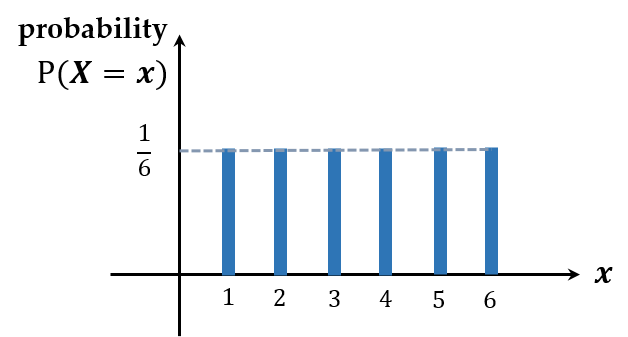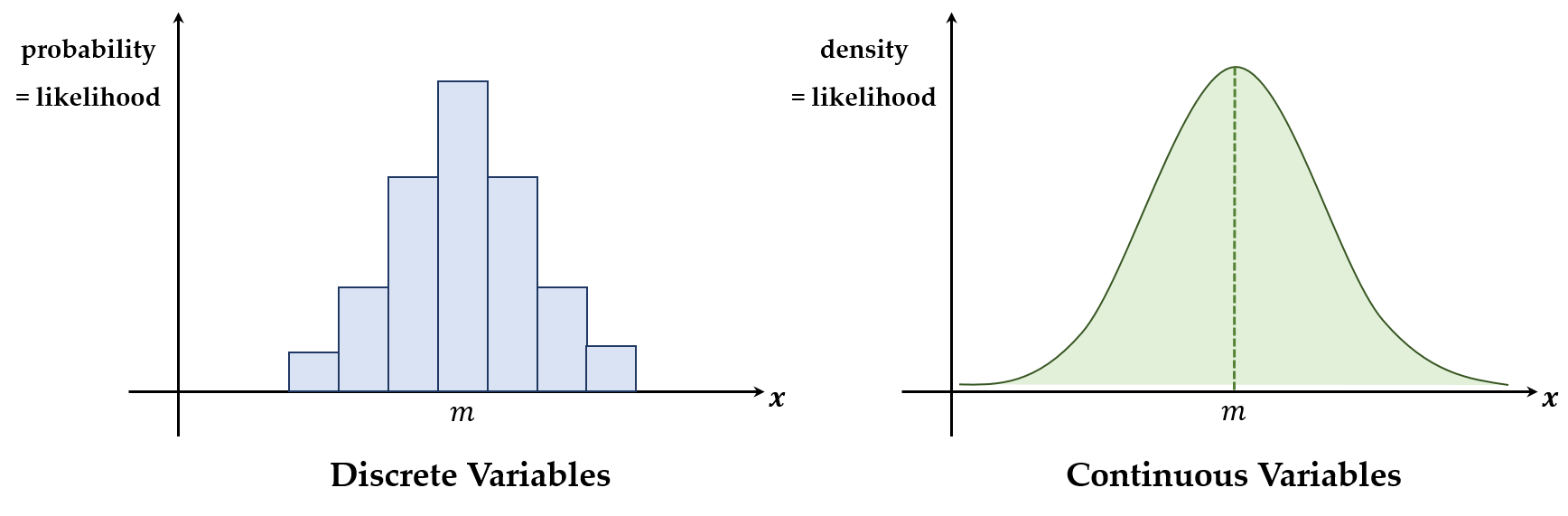# Probability and Likelihood

## 1. Discrete Variables

In the case that random variables have finite values. When the values a random variable $X$ can have are $x_1, \cdots, x_n$, the probability at $X = x_i$ is $p_i$ which is denoted by $P(X = x_i) = p_i$ for $1 \le i \le n$. Moreover, the function which consists of these probabilities $p_1, \cdots, p_n$ is called the probability mass function. For example, when rolling a dice, a random variable $X$ is a finite value and $p_i = \frac{1}{6}$. The probability mass function is constant as shown below.## 2. Continuous Variables

Consider the situation we select the number $5$ in $[1, 6]$. Unlike the case of rolling a dice, there are infinite numbers in this range, so the probatility of picking the number $5$ is $\frac{1}{\infty} = 0$. Other than this situation, picking the other real numbers has the same probability, $0$. Therefore, it is meaningless that we find the probability when we pick a number in a continuous range, so we should only consider the specific range. For example, when we pick a number in $[1, 6]$, the probability that the number is in $[4, 5]$ is $\frac{5 - 4}{6 - 1} = \frac{1}{5}$. This kind of probability functions cannot be represented by the probability mass function, and another probability function which is called the probability density function should be introduced. The area of this function in the specific range represents the probability that the number in this range is picked.As shown in the figure, the total area must be $\frac{1}{5} \times (6 - 1) = 1$ because it is the sum of all probabilites. Since the probability density function is continuous, the probability, which is the area in this case, can be calculated only in the specific range.

## 3. LikelihoodAs above, the shapes of functions look similar, and the probability at $m$ when a random variable $X$ is discrete is highest. However, when a random variable $X$ is continuous, the probability at $m$ is $0$. Although the events near $m$ are intuitively most likely happen, the probability is always $0$. This is an awkward situation, so another conept should be introduced which is called likelihood. As shown in the figure above, the likelihood has the different meaning depending on whether a random variable $X$ is discrete or continuous. However, it always means the $y$-axis value of the probability mass function or the probability density function. Note that the probability is the likelihood when $X$ is discrete, but is not when $X$ is continuous.